## Electricity and Magnetism

### Maxwell’s Equations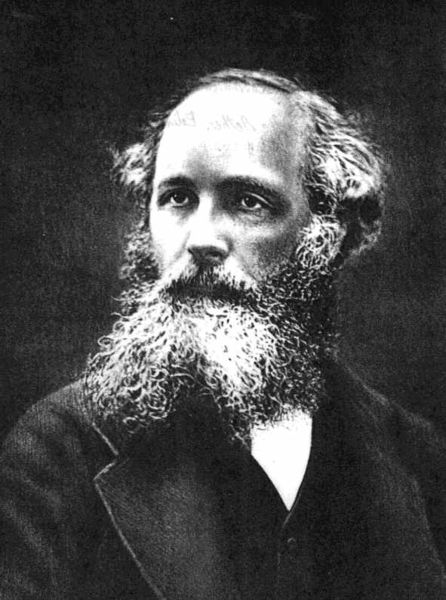James Clerk Maxwell’s facial hair resembling field lines of both electricity and magnetism sparked his unification of the electric and magnetic forces.
Source

Before the days of the Scottish mathematical physicist James Clerk Maxwell, electricity and magnetism weren’t together-together in the eyes of physicists. Physicists just thought electricity and magnetism flirted with one another, but that was it. It took the mathematical prowess of Maxwell to find out about this union. Einstein, himself with electric-field-line-emulating hair, called Maxwell’s work the “most profound and the most fruitful that physics has experienced since the time of Newton.” High praise indeed.

Since all Maxwell’s work is in vector calculus, we won’t have to memorize his four equations or use them, but four equations there are and they relate electricity and magnetism like Ampere’s Law, Guass’ law, Faraday’s Law, and Biot-Savart Law.

Here they are, in all their unintelligible-without-vector-calculus glory, for our head-scratching wonder and admiration, cue the choir of angels:Source

Fun Fact: The upside-down triangle is a vector operator called “del” or “nabla.” The top line would read “del dot D,” the last one “del cross H,” etc. The dots indicates “divergence,” a calculus operation, and the crosses indicate “curl,” which is a different calculus operation.

The equation on top is about electric flux, turning into Gauss’ Law. The second one says that there’s no flux with magnetic fields because of their two-pole nature. The third incorporates Faraday’s Law of Induction, and the last one is Ampere’s circuital Law. It doesn’t look like this in vector calculus form, but trust us, no one want’s to see the math.

### Electromagnetic Waves

It may not seem so to us without an understanding of vector calculus, but Maxwell’s equations reveal something fundamental.

Now, hold on to that thought. Before we expose what these equations reveal, we need two definitions. We need to know the differences and similarities of permittivity and permeability.

If an electric field forms in some type of medium, how much resistivity does it encounter? Permittivity is a measure of the inclination that some medium will have when presented with the challenge of forming a electric field. We write this out as ε = ε0εr, where ε is the total permittivity, ε0 is the permittivity in free space, and εr is the relative permittivity of the medium.

Permeability, μ0, on the other hand,is a bit like permittivity but in the world of magnetism instead of electric fields. Permeability is a measure of the inclination a type of medium will have when presented with the challenge of forming a magnetic field.

Back to Maxwell’s equations: the equations for E and B share the same form as wave propagation when in calculus form, which means that from these equations we—who are we kidding, the scientists— find the speed of electric and magnetic waves. Without further ado, it’s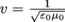.

We’ve seen that the permittivity constant ε0 has a value of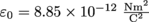,and that the permeability of free space µo has a value of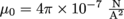. Solving for v yields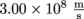.This number seems familiar somehow.…

That’s right—it’s the speed of light. What does it mean? Could it be that light is an electromagnetic wave? We’re all gasping and gaping, mouths open wide, jaws on the floor.
The speed limit for all light in the universe is determined by constants from electricity and magnetism. Light has both electric and magnetic fields.

Scientists definitely didn’t see that coming.

Maxwell might not have seen it coming either, but he recognized what it meant, and has Einstein’s admiration for it.

### Plane Waves

We know that the electromagnetic spectrum is composed of light of different frequencies or wavelengths. Let’s say our waves are plane waves all traveling in the z direction and are independent of x and y. Got it? Good.

Solutions to the three dimensional wave equations result in the wave equations E(z, t) = E0ei(kzwt) and B(z, t) = B0ei(kz wt) where E0 and B0 are the complex amplitudes of the electromagnetic waves. Alternatively, we may express the wave equations as Ez = E0 cos(ωtkz) and Bz = B0 cos(ωtkz) for a wave propagating in the z direction, or we could interchangeably use the sine function—all that’s required to model a wave is periodic behavior.

Reminders: in these two equations, k is the wave number, related to the wavelength, λ, with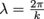.The angular frequency w is given by w = 2πf where f is the frequency of the wave. Remember frequency is the inverse of the period (T), so it gets to join the fun too.

Electromagnetic waves are transverse, meaning their displacement or oscillation is perpendicular to the direction of propagation. We define a polarization vector describing the wave’s plane of vibration in the direction of its electric field by convention. Since our waves are defined as traveling in the z direction, the waves may be polarized in either the x- or y-directions, or some combination of the two.

Here’s the picture worth all the words above: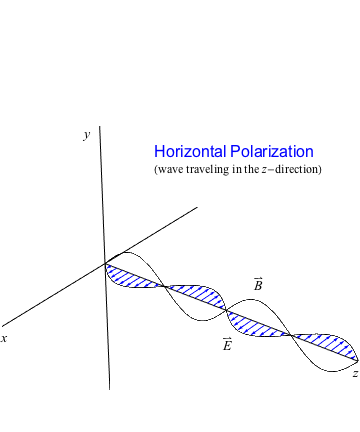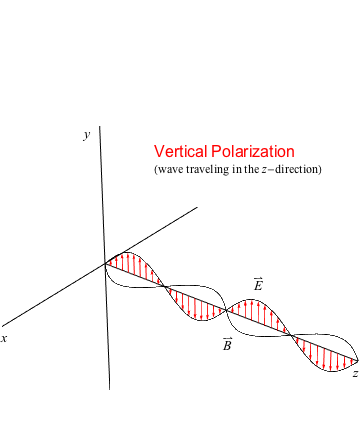In terms of our electromagnetic wave traveling at the speed of light, c, our electric field E and magnetic field B make up the two components of a transverse wave. As we see for each polarization, if E oscillates on the x-axis, then B is on the y-axis and vice versa. Both waves propagate in the z direction. It’s kind of crazy that light, without a charge itself—or mass, for that matter—could have electric and magnetic fields associated with it, but there we have it. The entire electromagnetic spectrum—from gamma rays down to radio waves—have these same properties, differing only in wavelength and frequency, an interchangeable statement since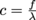.

### Common Mistakes

We’re not doing much math in this section, so it’s the concepts we need to double check.

### Brain Snack

The idea of electricity and magnetism having a close relationship first arose in 1820. It was a Danish scientist, Hans Christian Oersted, playing around with a compass. He discovered that an electric current would cause a deflection in the needle.